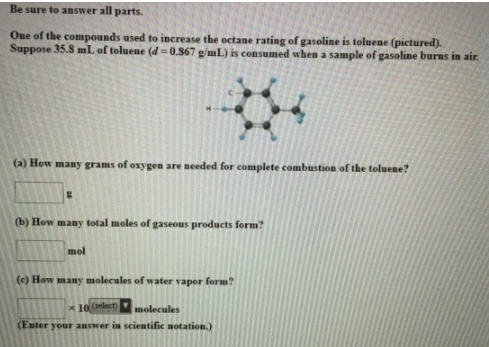# Problem: Be sure to answer all parts. One of the compounds used to increase the octane rating of gasoline is toluene (pictured). Suppose 35.8 mL of toluene (d = 0.867 g/mL) is consumed when a sample of gasoline burns in air. (a) How many grams of oxygen are needed for complete combustion of the toluene? (b) How many total moles of gaseous products form? (c) How many molecules of water vapor form? (Enter your answer in scientific notation.)

🤓 Based on our data, we think this question is relevant for Professor Eddleton's class at VT.

###### FREE Expert Solution###### Problem Details

Be sure to answer all parts.

One of the compounds used to increase the octane rating of gasoline is toluene (pictured). Suppose 35.8 mL of toluene (d = 0.867 g/mL) is consumed when a sample of gasoline burns in air.

(a) How many grams of oxygen are needed for complete combustion of the toluene?

(b) How many total moles of gaseous products form?

(c) How many molecules of water vapor form?